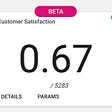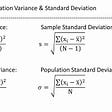# TV shows on Netflix, Prime Video, Hulu and Disney+ : A Data Analysis

`!pip install opendatasets — upgrade — quietdataset_url = ‘https://www.kaggle.com/ruchi798/tv-shows-on-netflix-prime-video-hulu-animport opendatasets as odod.download(dataset_url)data_dir = ‘./tv-shows-on-netflix-prime-video-hulu-and-disney’import pandas as pdimport numpy as npdf = pd.read_csv(‘./tv-shows-on-netflix-prime-video-hulu-and-disney/tv_shows.csv’)df.head()`
`df['Age'].fillna(value = '16+', inplace = True)df['IMDb'].fillna(value = 7.3,inplace = True)`
`df.drop('Rotten Tomatoes', axis = 1,inplace=True)df.drop('type',axis = 1, inplace = True)`
`df.info()df.describe()`
`import seaborn as snsimport matplotlibimport matplotlib.pyplot as plt%matplotlib inlinesns.set_style('darkgrid')matplotlib.rcParams['font.size'] = 14matplotlib.rcParams['figure.figsize'] = (9, 5)matplotlib.rcParams['figure.facecolor'] = '#00000000'`
`sns.distplot(df['Year'])`
`top_imdb_rated = df[['Title','IMDb']]top_imdb_rated = top_imdb_rated.sort_values(by = 'IMDb',ascending=False)[:10]top_imdb_ratedsns.barplot(x='IMDb', y = 'Title',data = top_imdb_rated)xtop_imdb_rated = df[['Title','IMDb']]xtop_imdb_rated = xtop_imdb_rated.sort_values(by = 'IMDb', ascending = True)[:10]xtop_imdb_rated`
`age = df['Age'].value_counts()ageage.plot.pie(x='Age',autopct='%1.2f%%')`
`platforms = df[[‘Netflix’, ‘Hulu’, ‘Prime Video’, ‘Disney+’]].apply(pd.Series.value_counts).reset_index()platforms = platforms.Tplatforms.drop(‘index’,inplace=True)platformsplatforms.plot.bar()`
`ratingdic = {}for i in ['Netflix', 'Hulu', 'Prime Video', 'Disney+']:      ratingdic['r_'+i] = (df[df[i]==1].IMDb.sum())/(df[df[i]==1][i].sum())rating = pd.DataFrame.from_dict(ratingdic,orient='index',columns=['Rating'])ratingsns.barplot(x='Rating', y =rating.index, data = rating)rating.plot.line()`
`platform_agedict = {}for i in [‘Netflix’, ‘Hulu’, ‘Prime Video’, ‘Disney+’]:      platform_agedict[‘a_’+i] = df[df[i]==1].Age.value_counts()platform_age = pd.DataFrame.from_dict(platform_agedict,orient='index')platform_age.fillna(0,inplace = True)platform_ageplatform_age.plot.bar()`
`platform_aget = platform_age.Tfig = plt.figure()ax1 = fig.add_axes([0, 0, 0.5, 0.5], aspect = 1)ax1.set_title('Age of Disney+')ax1.pie(platform_aget['a_Disney+'], labels = platform_aget.index,autopct='%1.1f%%')ax2 = fig.add_axes([0.3, 0, 0.5, 0.5], aspect = 1)ax2.set_title('Age of Hulu')ax2.pie(platform_aget['a_Hulu'], labels = platform_aget.index,autopct='%1.1f%%')ax3 = fig.add_axes([0.6, 0, 0.5, 0.5], aspect = 1)ax3.set_title('Age of Netflix')ax3.pie(platform_aget['a_Netflix'], labels = platform_aget.index,autopct='%1.1f%%')ax4 = fig.add_axes([0.9, 0, 0.5, 0.5], aspect = 1)ax4.set_title('Age of Prime Video')`
`yearwise = {}for i in [‘Netflix’, ‘Hulu’, ‘Prime Video’, ‘Disney+’]:     yearwise[i+’_year’] = df[df[i] == 1][‘Year’].value_counts()dfyearwise = pd.DataFrame.from_dict(yearwise,orient='index')dfyearwise.fillna(0,inplace = True)dfyearwise['Netflix_year'].max()dfyearwise['Hulu_year'].max()dfyearwise['Prime Video_year'].max()dfyearwise['Disney+_year'].max()`

# References and Future Work

--

--

--

## More from Aanand S

Under the sky. Love everything

Love podcasts or audiobooks? Learn on the go with our new app.

## Data Science from a beginner’s side..## The Data Scientist From 2018 To 2020: What Has Changed?## Blending skills with backgrounds## How to predict customer satisfaction over chat?## Exploratory Data Analysis Web Application## Fine-Tuning Language Models the Easy Way with blather## Partial Correlation Vs. Conditional Mutual Information## Practicum Spotlight## Aanand S

Under the sky. Love everything

## Missing Values Identifications in Tabular Data## Foundations of Statistics for learning Data Science## Working with missing data on Pandas